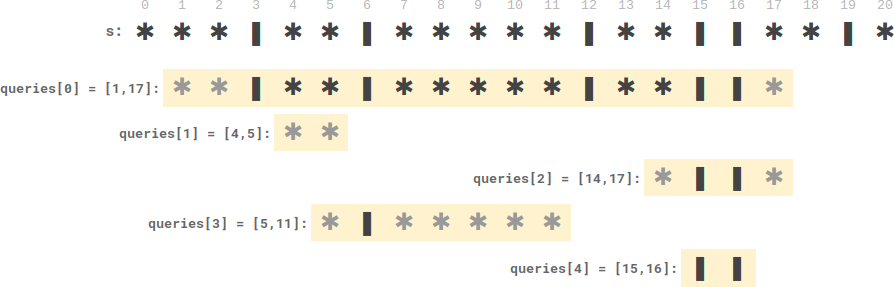| English | 简体中文 |

# 2055. Plates Between Candles

## Description

There is a long table with a line of plates and candles arranged on top of it. You are given a 0-indexed string s consisting of characters '*' and '|' only, where a '*' represents a plate and a '|' represents a candle.

You are also given a 0-indexed 2D integer array queries where queries[i] = [lefti, righti] denotes the substring s[lefti...righti] (inclusive). For each query, you need to find the number of plates between candles that are in the substring. A plate is considered between candles if there is at least one candle to its left and at least one candle to its right in the substring.

• For example, s = "||**||**|*", and a query [3, 8] denotes the substring "*||**|". The number of plates between candles in this substring is 2, as each of the two plates has at least one candle in the substring to its left and right.

Return an integer array answer where answer[i] is the answer to the ith query.

Example 1:Input: s = "**|**|***|", queries = [[2,5],[5,9]]
Output: [2,3]
Explanation:
- queries has two plates between candles.
- queries has three plates between candles.


Example 2:Input: s = "***|**|*****|**||**|*", queries = [[1,17],[4,5],[14,17],[5,11],[15,16]]
Output: [9,0,0,0,0]
Explanation:
- queries has nine plates between candles.
- The other queries have zero plates between candles.


Constraints:

• 3 <= s.length <= 105
• s consists of '*' and '|' characters.
• 1 <= queries.length <= 105
• queries[i].length == 2
• 0 <= lefti <= righti < s.length